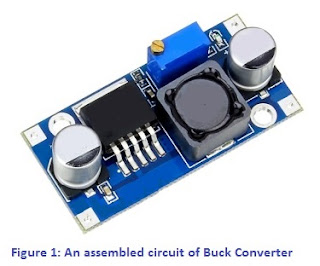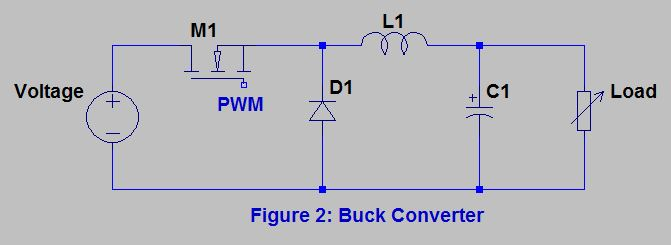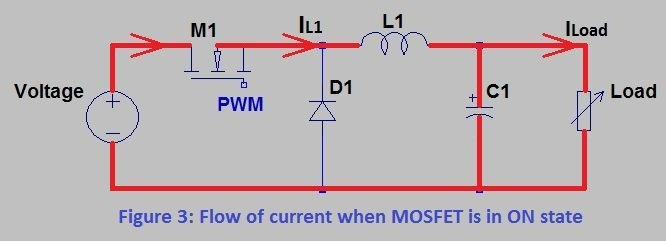# Buck Converter

### What is a Buck Converter?

A buck converter can be define as, a converter circuit which steps down the output voltage by increasing output current from the applied input voltage is called as DC to DC buck converter.

Let’s see some buck converter basicsIt is a type of Switch Mode Power Supply as it uses a diode and a transistor (or MOSFET) as a switching semiconductor device. Buck converter results in high efficiency as compare to linear regulator circuits which has losses in the form of heat.

Sometimes for advance level we can use a transistor in place of diode for synchronous rectification.

Along with these semiconductor devices buck converter circuit contains energy storage passive components like capacitor and inductor. These components will help to reduce voltage ripples at the output load side.

Figure 1 shows an assembled circuit of Buck Converter.An assembled circuit of Buck Converter

### Buck Converter Working

Please refer below figure 2 for the buck converter circuit diagram;Buck Converter
Circuit consists of;
• MOSFET (M1) which acts as a switching element and turns ON and OFF.
• Driver IC (PWM) which generates PWM signal to the Gate pin of MOSFET M1. This driver IC controls the MOSFET ON and OFF by the programmed duty cycle. This driver IC or control IC uses a closed feedback loop to regulate the output voltage.
• Inductor (L1) and Capacitor (C1) acts as a low pass filter which gives ripple free DC voltage at output.
• Diode (D1) is a flywheel diode and act as a closed loop to allow the current in the inductor (L1) to flow to the load.
Buck converter operation can be understood by the flow of current and voltage in the circuit. Below working steps clear this;

• When the MOSFET is in OFF state, there is no current in the circuit (I considered MOSFET as a switch).
• When the MOSFET goes first time in ON state, the current will starts to increase, and because of this inductor will generates an opposing voltage through its terminals.
• This voltage drop offsets the voltage of the source and this result in reduction of the remaining voltage across the load.
• With the time the amount of variation of current decreases and results in decrease of voltage across the inductor. This increases voltage at the load. With this time, energy i.e. magnetic field stored in the inductor.
• If the MOSFET is in OFF state and there is moving current, then it results in a voltage drop through the inductor, therefore the remaining voltage at the output will always be less than the input source voltage.
• Now the MOSFET is in OFF state, there will be no source voltage and this result in decrease of current.
• This decrease in current produces a voltage drop through the inductor. This voltage drop will be opposite to the resultant voltage drop when the MOSFET is in ON state. This results inductor to develop as a Current Source.
• Energy is stored in the inductor as magnetic field which results in the flow of current through the load.
• When the input source voltage is disconnected this current still flows, this current adds with the current which flows when MOSFET is ON state. It results in current which is greater than the average input current (which becomes zero when MOSFET is in OFF state).
• So, average increase of current results in reduction of voltage and preferably conserves the power supplied to the load.
• During the MOSFET OFF state, the inductor discharges its stored energy into the rest of the circuit. If the MOSFET ON again before the inductor fully discharges, the load voltage will be always greater than zero.

Red path in figure 3 at below shows; flow of current when MOSFET is in ON state.Flow of current when MOSFET is in ON state
Red path in figure 4 at below shows; flow of current when MOSFET is in OFF state.Flow of current when MOSFET is in OFF state
Now let’s see how diode D1 works in the circuit;
• Here the flywheel diode provides the current pathway when the MOSFET turns off.
• When we make the MOSFET ON, the inductor current flows in the forward direction providing the load current and charging the capacitor. Little amount of energy is also stored in inductor.
• When MOSFET turns OFF, inductor does not allow the current to reduce to zero.
• As the voltage source is cut-off from the circuit, charged capacitor supplies little current through this diode.
• Also, energy stored in inductor freewheels through this diode and provides current till the effect of inductor is cancelled out.
• In simple words when MOSFET is ON, the diode acts as reverse biased and when MOSFET is OFF the diode acts as forward biased.
• When D1 reverse biased capacitor C1 charges and when D1 forward biased capacitor C1 discharges.

### Buck Converter Applications

Below are some of the applications of Buck Converter;

• SMPS
• Solar chargers
• Battery Chargers in Automobiles
• Audio Amplifiers
• Brushed Motor Controllers
and there are many more….

### Conclusion

We have seen the definition of Buck Converter, its basic circuit diagram, role of components, its working and some of its application.

Buck converter is most commonly used converter topology as its uses switching elements and driver IC, which generates duty cycles and which works in closed feedback loop to regulate the output voltage.

Kozmotronik
AUTHOR

Thanks. It's a good articleAUTHORUnknown
AUTHOR

Good keep it up